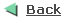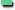OLAP DB-Design with DimensionsTerminology The following clarifies some basic data warehousing (OLAP) terms: Dimension tables describe the business entities of an enterprise, which usually represent hierarchical, categorical information such as time, departments, locations, and products. Dimension tables are sometimes called lookup or reference tables. Dimension tables usually change slowly over time and are not modified on a periodic schedule. They are typically not large, but they affect the performance of long-running decision support queries that consist of joins of fact tables with dimension tables, followed by aggregation to specific levels of the dimension hierarchies. Fact tables describe the business transactions of an enterprise. Fact tables are sometimes called detail tables. The vast majority of data in a data warehouse is stored in a few very large fact tables. They are updated periodically with data from one or more operational online transaction processing (OLTP) databases. Fact tables also contain one or more keys that organize the business transactions by the relevant business entities such as time, product, and market. In most cases, the fact keys are non-null, form a unique compound key of the fact table, and join with one and only one row of a dimension table. A materialized view is a pre-computed table comprising aggregated and/or joined data from fact and possibly dimension tables. Builders of data warehouses will know a materialized view as a summary or aggregation. Example A telephone company registers all voice calls in a fact table, so called "CALL". The corresponding voice numbers (MSISDNs) are stored in the table "MSISDN". Voice calls can be located in an area, which is located in a country. For statistic reasons, the denormalized table "STAT" has been built, this table represents a typical hierarchiy between day, week, month and year. The dimension "STATDIM" is build over this statistic table.1. Create the Tables CREATE TABLE country (   ctr_code    NUMBER(10)      PRIMARY KEY,   ctr_name    VARCHAR2(20)    NOT NULL ); CREATE TABLE area (   area_code     NUMBER(10) PRIMARY KEY,   area_name     VARCHAR2(20) NOT NULL,   ctr_code      NUMBER(10) REFERENCES country(ctr_code) ); CREATE TABLE msisdn (   msn_id   NUMBER(10) PRIMARY KEY,   msisdn   VARCHAR2(20) NOT NULL,   status   VARCHAR2(20) NOT NULL ); CREATE TABLE call (    call_id     NUMBER(10)      NOT NULL PRIMARY KEY,    call_date   DATE            NOT NULL,    msn_id      NUMBER(10)      NOT NULL REFERENCES msisdn(msn_id),    amount      NUMBER(7,2)     NOT NULL,    area_code   NUMBER(10)      NOT NULL REFERENCES area(area_code) ); 2. Create denormalized statistic table The denormalized statistic table "STAT" will be used by the dimension "STATDIM" to implement the hierarchy between day, week, month, etc. CREATE TABLE stat (   day           DATE PRIMARY KEY,   week          NUMBER(2)      NOT NULL,   month         NUMBER(2)      NOT NULL,   month_name    VARCHAR2(20)   NOT NULL,   quarter       VARCHAR2(20)   NOT NULL,   year          NUMBER(4)       NOT NULL,   season        VARCHAR2(20)   NOT NULL ); CREATE INDEX idx_stat_year on stat(year); ALTER TABLE call ADD CONSTRAINT call_date_stat_fk   FOREIGN KEY (call_date) REFERENCES stat(day); EXEC dbms_utility.analyze_schema('SCOTT','COMPUTE'); 3. Create the Dimension A dimension defines a parent-child relationship between pairs of column sets, where all the columns of a column set must come from the same table. However, columns in one column set (or "LEVEL") can come from a different table than columns in another set. The optimizer uses these relationships with materialized views to perform query rewrite. "HIERARCHY" defines a linear n:1 hierarchy of levels in the dimension. Each hierarchy forms a chain of parent-child relationships among the levels in the dimension. Hierarchies in a dimension are independent of each other. "ATTRIBUTE" specifies the columns that are uniquely determined by a hierarchy level to perform 1:1 relations. The hierarchy between dates as day, week, month and year are represented in the dimension "STATDIM". CREATE DIMENSION statdim   LEVEL day         IS stat.day   LEVEL week        IS stat.week   LEVEL month       IS stat.month   LEVEL quarter     IS stat.quarter   LEVEL season      IS stat.season   LEVEL year        IS stat.year HIERARCHY calendar_hierarchy (   day         CHILD OF   month       CHILD OF   quarter     CHILD OF   year) HIERARCHY season_hierarchy (   day      CHILD OF   season   CHILD OF   year) HIERARCHY week_hierarchy (   day      CHILD OF   week     CHILD OF   year) ATTRIBUTE month DETERMINES month_name; 4. Check the integrity of the dimension EXEC dbms_olap.validate_dimension('STATDIM','SCOTT',FALSE,TRUE); SELECT table_name,        dimension_name,        relationship,        bad_rowid FROM mview\$_exceptions; No rows are found in the exception table, so the dimension hierarchy is setup correctly. 5. Create the MVIEW DROP MATERIALIZED VIEW call_sum; CREATE MATERIALIZED VIEW call_sum ENABLE QUERY REWRITE AS SELECT msisdn.msisdn, area.area_name, stat.month, SUM(call.amount) AS total_call       FROM call, area, stat, msisdn      WHERE call.area_code = area.area_code        AND call.call_date = stat.day        AND call.msn_id    = msisdn.msn_id   GROUP BY msisdn.msisdn, area.area_name, stat.month; ANALYZE TABLE call_sum COMPUTE STATISTICS; 6. Test the query with the execution plan The query collects the amount of calls grouped by year, not by month as defined in the MVIEW. Although the query rewrite works, because the optimizer knows the hierarchy, thanks the dimension "STATDIM" as the execution plan shows. ALTER SESSION SET query_rewrite_integrity=TRUSTED; SET AUTOTRACE ON EXPLAIN SELECT msisdn.msisdn, area.area_name, stat.year, SUM(call.amount)       FROM call, area, stat, msisdn      WHERE call.area_code = area.area_code        AND call.call_date = stat.day        AND call.msn_id    = msisdn.msn_id   GROUP BY msisdn.msisdn, area.area_name, stat.year; Execution Plan ----------------------------------------------------------    0      SELECT STATEMENT Optimizer=CHOOSE (Cost=7 Card=15 Bytes=420)    1    0   SORT (GROUP BY) (Cost=7 Card=15 Bytes=420)    2    1     HASH JOIN (Cost=5 Card=25 Bytes=700)    3    2       VIEW (Cost=3 Card=3 Bytes=15)    4    3         SORT (UNIQUE) (Cost=3 Card=3 Bytes=15)    5    4            TABLE ACCESS (FULL) OF 'STAT' (Cost=1 Card=8 Bytes=40)    6    2       TABLE ACCESS (FULL) OF 'CALL_SUM' (Cost=1 Card=33 Bytes=759) Dimension between Tables In the example above, we used a dimension "STATDIM" which is based on one certain table, in this case "STAT". However it is possible to extend the dimension to more than one table using the JOIN KEY clause in the CREATE DIMENSION statement. There is a hierachy between county and area; a country may have zero, one or more areas, one area must be located in a country. We want to build a dimension over these two tables as well. 1.  Create Dimension over two tables CREATE DIMENSION areadim   LEVEL area_code   IS area.area_code   LEVEL area_name   IS area.area_name   LEVEL ctr_code    IS country.ctr_code   LEVEL ctr_name    IS country.ctr_name HIERARCHY area_hierarchy (   area_code   CHILD OF   ctr_code    JOIN KEY area.ctr_code REFERENCES ctr_code) ATTRIBUTE area_code DETERMINES area.area_name ATTRIBUTE ctr_code DETERMINES country.ctr_name; 2. Create MVIEW with columns from both tables DROP MATERIALIZED VIEW call_sum; CREATE MATERIALIZED VIEW call_sum ENABLE QUERY REWRITE AS SELECT msisdn.msisdn, country.ctr_code,            area.area_name, stat.month, SUM(call.amount) AS total_call       FROM call, area, stat, msisdn, country      WHERE area.ctr_code = country.ctr_code        AND call.area_code = area.area_code        AND call.call_date = stat.day        AND call.msn_id    = msisdn.msn_id   GROUP BY msisdn.msisdn, country.ctr_code, area.area_name, stat.month; ANALYZE TABLE call_sum COMPUTE STATISTICS; 6. Test the query with the execution plan SELECT msisdn.msisdn, country.ctr_code,        area.area_name, stat.year, SUM(call.amount)       FROM call, area, stat, msisdn, country      WHERE area.ctr_code = country.ctr_code        AND call.area_code = area.area_code        AND call.call_date = stat.day        AND call.msn_id    = msisdn.msn_id   GROUP BY msisdn.msisdn, country.ctr_code, area.area_name, stat.year; Execution Plan ----------------------------------------------------------    0      SELECT STATEMENT Optimizer=CHOOSE (Cost=7 Card=22 Bytes=660)    1    0   SORT (GROUP BY) (Cost=7 Card=22 Bytes=660)    2    1     HASH JOIN (Cost=5 Card=25 Bytes=750)    3    2       VIEW (Cost=3 Card=3 Bytes=15)    4    3         SORT (UNIQUE) (Cost=3 Card=3 Bytes=15)    5    4            TABLE ACCESS (FULL) OF 'STAT' (Cost=1 Card=8 Bytes=40)    6    2       TABLE ACCESS (FULL) OF 'CALL_SUM' (Cost=1 Card=33 Bytes=825) Download Example: Click here More Information Another example about dimensions can be found here.